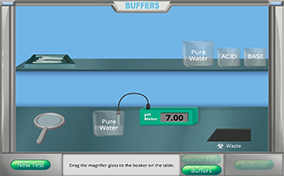# BuffersMost organisms cannot survive if their pH changes outside of a very limited range. For instance, humans' arterial blood has a normal pH range of 7.35–7.45. Changes outside of this range can lead to serious complications. If the pH goes below 6.80 or above 7.80, the body's cells can stop functioning, resulting in death. How does a human body maintain a constant pH even when exposed to acidic or alkaline substances? The answer is buffers. In this investigation, find out about what buffers are and how they work.

### What is an acid?

An acid is a substance that typically has the following characteristics:
• forms the hydronium ion (H3O+) when placed in water
• donates the hydrogen ion (H+)
• accepts electrons
• tastes sour
• has a pH less than 7
• reacts with active metals
• reacts with carbonates

### What is a base?

A base is a substance that typically has the following characteristics:
• forms the hydroxide ion (OH-) when placed in water
• accepts the hydrogen ion (H+)
• donates electrons
• tastes bitter
• has a pH greater than 7
• feels slippery

### What is pH?

The power of hydrogen is represented by the symbol, pH. The pH is a measure of the acidity or alkalinity of a solution. The scale generally ranges from 0–14, with 7 being the neutral point. Values of pH less than 0 and greater than 14 are possible, but are rarely encountered in typical laboratory experiences. The formula to calculate pH is given by: pH = -log [H+] Please note that the hydrogen ion (H+) is highly solvated in water and quickly forms the hydronium ion [H3O+]. Many textbooks show the pH equation as: pH = -log [H3O+] Both equations are technically correct and will yield the same result for simple acid-base problems.

### What does a neutral pH mean?

A neutral pH indicates that the concentrations of the [H+] and [OH-] are equal. The pH value of a neutral system is 7.00. This shows that the [H+] and [OH-] are both equal to 1.00 x 10-7. Pure water has a neutral pH. If the solution is basic, the [OH-] is greater than the [H+]. If the solution is acidic, then the [H+] is greater than the [OH-].

### What is the function of a buffer?

A buffer is a two-part chemical system that, together, prevents changes in a solution’s pH. Normally, when an acid is added to a system, the pH decreases. Likewise, if a base is added to a system, the pH is expected to increase. A buffer is a system that resists changes in pH upon the addition of relatively small amounts of an acid or a base.

### How is a buffer made?

A buffer is made by combining large amounts of a weak acid with large amounts of the acid’s conjugate base. If your chemical common sense is working, the previous statement should cause you concern. By definition, a weak acid produces very small amounts of its conjugate base. So how can the system contain both the weak acid and large amounts of its conjugate base? The conjugate base is added to the system in the form of a soluble salt and does not come from the breakdown of the weak acid. A buffer can also be made by combining large amounts of a weak base with large amounts of the base’s conjugate acid.

### How do buffers work?

Buffers work by converting excess hydrogen ions (H+1) or hydroxide ions (OH-1) added to the solution (which would normally change the pH) into a chemical form that will not change the pH. The two parts of a buffer system are called the conjugate base and the conjugate acid.
• The conjugate base will accept hydrogen, reacting with excess hydrogen ions to become the conjugate acid.
• The conjugate acid can donate a hydrogen, reacting with excess hydroxide ions to be converted into the conjugate base.
For example, carbonic acid (H2CO3) and its conjugate base, the bicarbonate ion (HCO3-) make up one buffered system. If an acid is added to the system, the bicarbonate ion grabs the H+ added from the acid and converts to carbonic acid. Since the concentration of [H+] does not change, the pH remains the same. Likewise, if base is added to the buffered system, the carbonic acid donates a hydrogen ion that reacts with the excess hydroxide ions (OH-) forming water and the bicarbonate ion. Again, this results in the pH remaining relatively constant.

### What is the dissociation constant for an acid?

The acid dissociation constant (Ka) is a measure of the strength of the acid. The larger the value of Ka, the more likely the acid is to donate hydrogen ions and "split" apart into the conjugate base. A strong acid, like hydrochloric acid (HCl) would have a large Ka value, while a weak acid like hydrofluoric acid (HF) would have a small value, much less than one (1). To make Ka’s interpretations easier, many times the logarithmic Ka value is used called pKa. The pKa is the determined using the following equation: pKa = -log(Ka) As the value of pKa increases, the strength of an acid decreases and indicates a smaller dissociation of the acid. As the value of pKa decreases, the strength of an acid increases and indicates a greater dissociation of the acid.

### How is the logarithmic dissociation constant for a buffered system calculated?

If the pH and ratio of conjugate base to weak acid of a buffered system is known, the dissociation constant for the system can be determined using the following equation: Dissociation Constant (pKa) = pH – log ([A-1]/[HA]) Where the dissociation constant is pKa, [A-] is the concentration of the conjugate base, and [HA] is the concentration of the weak acid.